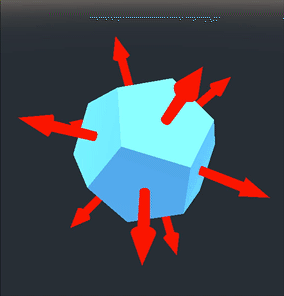# KinematicBody: align with surface

## Problem

You need your kinematic body to align with the surface or terrain.

## Solution

This recipe builds on the basic `KinematicBody` controller described in the KinematicBody: Movement recipe, so read that one first.

First, we’ve added some terrain to the scene. You can download the terrain from here: https://fertile-soil-productions.itch.io/modular-terrain-pack. This is low-poly terrain, but you can use or make any terrain you like for this technique.

As you can see, the movement still works with the terrain, but the tank seems to “float” above the slopes because it doesn’t change its orientation.

Instead, we need to rotate the tank so that its treads are aligned with the ground, even as the slope changes. To do that, we need to know which way is up.

### Surface normals

A surface normal is a unit vector (“normal vector” and “unit vector” mean the same thing) perpendicular to a surface. It shows which way the surface is facing. In the case of a mesh, every surface has a normal pointing outward.In Godot, when a body collides, you can get the normal of the collision. This will be the colliding body’s normal at the point of contact.

Once we have the surface normal, we need to align the tank’s Y axis with it. Note that we can’t use `Transform.looking_at()`, because that will align the -Z (forward) axis with the normal.

To do this, we’ll use the following function:

``````func align_with_y(xform, new_y):
xform.basis.y = new_y
xform.basis.x = -xform.basis.z.cross(new_y)
xform.basis = xform.basis.orthonormalized()
return xform
``````

Given a transform and a new Y direction vector, this function returns the transform rotated so that its `basis.y` is aligned with the given normal.

We can update the tank’s movement code to call this function when it collides with a surface:

``````func _physics_process(delta):
velocity += gravity * delta
get_input(delta)
velocity = move_and_slide_with_snap(velocity, Vector3.DOWN*2, Vector3.UP, true)
for i in get_slide_count():
var c = get_slide_collision(i)
global_transform = align_with_y(global_transform, c.normal)
``````

This doesn’t work quite as expected:

The problem is that the tank’s collision shape could be colliding with more than one of the terrain’s faces. Also, `move_and_slide()` can result in more than one collision in a single frame. This leads to the jittering. We need to choose one face and stick with it.

Add a `RayCast` child to the tank and set its Cast To to `(0, -2, 0)`. Don’t forget to check the Enabled property.

Since this raycast is pointing down from the exact center of the tank, we’ll align with the individual surface that it collides with - the one directly beneath the tank.

``````func _physics_process(delta):
velocity += gravity * delta
get_input(delta)
velocity = move_and_slide_with_snap(velocity, Vector3.DOWN*2, Vector3.UP, true)
var n = \$RayCast.get_collision_normal()
global_transform = align_with_y(global_transform, n)
``````

This is much better, but because we are instantly snapping to the new alignment every time the tank crosses an edge, it still looks a little jarring:

We can solve this last problem by interpolating to the new transform rather than snapping immediately to it.

``````func _physics_process(delta):
velocity += gravity * delta
get_input(delta)
velocity = move_and_slide_with_snap(velocity, Vector3.DOWN*2, Vector3.UP, true)
var n = \$RayCast.get_collision_normal()
var xform = align_with_y(global_transform, n)
global_transform = global_transform.interpolate_with(xform, 0.2)
``````

The result is much smoother and more pleasing:

Feel free to experiment with the interpolation amount. We found `0.2` to work well in this situation, but you might find a higher or lower value works better for you.